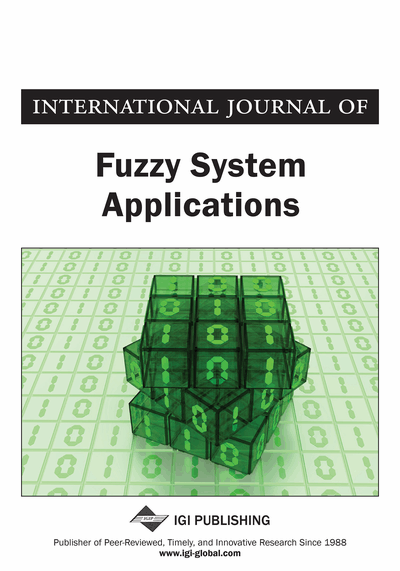# Developing a New Approach to Solve Solid Assignment Problems Under Intuitionistic Fuzzy Environment

P. Senthil Kumar
DOI: 10.4018/IJFSA.2020010101
OnDemand:
(Individual Articles)
Available
\$37.50
No Current Special Offers

## Abstract

When people solve real-life SAP they tend to face the uncertainty state as well as hesitation due to many uncontrollable factors. To deal with uncertainty and hesitation many authors have suggested the intuitionistic fuzzy representation for the data. In this article, the author tried to categorise the SAP under uncertain environment. He formulates the IFSAP and utilizes the TIFN to deal with uncertainty and hesitation. The SAP has uncertainty and hesitation in cost/time/profit/production is known as FIFSAP. The PSK (P. Senthil Kumar) method for finding an optimal solution for FIFAP is extended to solve the FIFSAP and the optimal objective value of FIFSAP is obtained in terms of TIFN. The main advantage of this method is that the optimal solution/assignment of FIFSAP is obtained without using the Hungarian method and intuitionistic fuzzy reduction method. Moreover, the proposed method is computationally very simple and easy to understand. The numerical example is presented to demonstrate computing procedure. The results affirm efficiency of the proposed method.
Article Preview
Top

## 1. Introduction

Assignment problem (AP) is a special type of a transportation problem (TP) in which the aim of the decision maker (DM) is to assign n number of machines to n number of jobs at a minimum time / minimum cost / maximum profit / maximum production. An assignment problem can be viewed as a transportation problem in which all supplies and demands equal to one. Assignment problem is used worldwide in solving real world problems. An assignment problem plays a vital role in assigning of accountants to accounts of the clients, trucks to drivers, contracts to bidders by systematic bid-evaluation, jobs to persons, routes to trucks, research teams to problems, sales/marketing people to sales territories, machines to operators, or police vehicles to patrolling areas, and so on.

An assignment problem has been used in a variety of application contexts such as resource allocation, manpower planning, personnel scheduling and so on. The standard assignment problem consists of assigning a number of tasks to an equal number of agents. Each agent is assigned to exactly one task and each task has exactly one agent assigned to perform it in such a way to minimize the overall cost (or time) of assigning agents to tasks. When the standard assignment problem is considered with the minimization of assignment cost as the objective function, it is called the cost minimizing assignment problem. The well- studied Hungarian method developed by Kuhn (1955) is recognized to be the first practical method for solving the standard two-dimensional assignment problem.

To solve the classical assignment problem using Hungarian method, one should know time of completion or cost of making all the possible assignments. Each assignment problem has a two-dimensional table, jobs (or tasks) one wishes to assign are represented in the rows and persons (or machines) to be assigned are expressed in the columns. Cost / time for each particular assignment is in the numbers in the two-dimensional table. Particularly, this table is referred as cost / time matrix of the two-dimensional assignment problem or classical assignment problem. Hungarian method is based on the principle that if a constant is added (or subtracted) to the elements of cost / time matrix, the optimum solution (or optimum assignment) of the assignment problem is the same as the original problem. Original cost / time matrix is reduced to another cost / time matrix by adding (or subtracting) a constant value to the elements of rows and columns of cost / time matrix where the total cost or total completion time of an assignment is zero. Further, this assignment is referred as the optimum assignment (or optimal assignment) since the optimum assignment remains unchanged after the reduction.

Let us consider n jobs J1, J2, …, Jn and n machines M1, M2, …, Mn (one machine to one job). Let cij be the unit cost of assigning ith machine to the jth job and let xij denotes that jth job is to be assigned to ith machine. Our objective is to determine the assignment of jobs to machines so that the total cost of completing all the jobs is minimum.

(3) where cij is the cost of assigning the job j to the machine i.

Now, the above problem can be put in the following assignment table (see Table 1).

## Complete Article List

Search this Journal:
Reset
Volume 13: 1 Issue (2024)
Volume 12: 1 Issue (2023)
Volume 11: 4 Issues (2022)
Volume 10: 4 Issues (2021)
Volume 9: 4 Issues (2020)
Volume 8: 4 Issues (2019)
Volume 7: 4 Issues (2018)
Volume 6: 4 Issues (2017)
Volume 5: 4 Issues (2016)
Volume 4: 4 Issues (2015)
Volume 3: 4 Issues (2013)
Volume 2: 4 Issues (2012)
Volume 1: 4 Issues (2011)
View Complete Journal Contents Listing